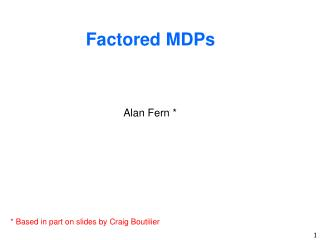DownloadDownload PresentationFactored MDPs

# Factored MDPs

Download Presentation## Factored MDPs

- - - - - - - - - - - - - - - - - - - - - - - - - - - E N D - - - - - - - - - - - - - - - - - - - - - - - - - - -
##### Presentation Transcript

1. Factored MDPs Alan Fern * * Based in part on slides by Craig Boutilier

2. Planning in Large State Space MDPs • You have learned algorithms for computing optimal policies • Value Iteration • Policy Iteration • These algorithms explicitly enumerate the state space • Often this is impractical • Simulation-based planning and RL allowed for approximate planning in large MDPs • Did not utilize an explicit model of the MDP. Only used a strong or weak simulator. • What if we had a compact representation for a large MDP and could efficiently plan with it? • Would allow for exact solutions to very large MDPs. • We will study representations and algorithms for doing this

3. A Planning Problem

4. Logical or Feature-based Problems • For most AI problems state are not viewed as atomic entities. • They contain structure. For example, they are described by a set of propositions • |S| exponential in number of propositions • Basic policy and value iteration do nothing to exploit the structure of the MDP when it is available

5. Solution? • Require structured representations • compactly represent transition function • compactly represent reward function • compactly represent value functions and policies • Require structured computation • perform steps of PI or VI directly on structured representations • can avoid the need to enumerate state space • We start by representing the transition structure as dynamic Bayesian networks

6. Propositional Representations • States decomposable into state variables • Structured representations the norm in AI • Decision diagrams, Bayesian networks, etc. • Describe how actions affect/depend on features • Natural, concise, can be exploited computationally • Same ideas can be used for MDPs

7. Robot Domain as Propositional MDP • Propositional variables for single user version • Loc (robot’s locat’n): Office, Entrance • T (lab is tidy): boolean • CR (coffee request outstanding): boolean • RHC (robot holding coffee): boolean • RHM (robot holding mail): boolean • M (mail waiting for pickup): boolean • Actions/Events • move to an adjacent location, pickup mail, get coffee, deliver mail, deliver coffee, tidy lab • mail arrival, coffee request issued, lab gets messy • Rewards • rewarded for tidy lab, satisfying a coffee request, delivering mail • (or penalized for their negation)

8. State Space • State of MDP: assignment to these six variables • 64 states • grows exponentially with number of variables • Transition matrices • 4032 parameters required per matrix • one matrix per action (6 or 7 or more actions) • Reward function • 64 reward values needed • Factored state and action descriptions will break this exponential dependence (generally)

9. Dynamic Bayesian Networks (DBNs) • Bayesian networks (BNs) a common representation for probability distributions • A graph (DAG) represents conditional independence • Conditional probability tables (CPTs) quantify local probability distributions • Dynamic Bayes net action representation • one Bayes net for each action a, representing the set of conditional distributions Pr(St+1|At,St) • each state variable occurs at time t and t+1 • dependence of t+1 variables on t variables depicted by directed arcs

10. T T(t+1) T(t+1) T 0.91 0.09 F 0.0 1.0 DBN Representation: deliver coffee RHM R(t+1) R(t+1) T 1.0 0.0 F 0.0 1.0 RHMt RHMt+1 Pr(RHMt+1|RHMt) Mt Mt+1 Pr(Tt+1| Tt) Tt Tt+1 L CR RHC CR(t+1) CR(t+1) O T T 0.2 0.8 E T T 1.0 0.0 O F T 0.1 0.9 E F T 0.1 0.9 O T F 1.0 0.0 E T F 1.0 0.0 O F F 0.1 0.9 E F F 0.1 0.9 Lt Lt+1 CRt CRt+1 RHCt RHCt+1 Pr(CRt+1 | Lt,CRt,RHCt)

11. RHMt RHMt+1 Mt Mt+1 Tt Tt+1 s1 s2 ... s64 Lt Lt+1 s1 0.9 0.05 ... 0.0 s2 0.0 0.20 ... 0.1 . . . S64 0.1 0.0 ... 0.0 CRt CRt+1 RHCt RHCt+1 Benefits of DBN Representation Pr(St+1 | St) = Pr(RHMt+1,Mt+1,Tt+1,Lt+1,Ct+1,RHCt+1 | RHMt,Mt,Tt,Lt,Ct,RHCt) = Pr(RHMt+1 |RHMt) * Pr(Mt+1 |Mt) * Pr(Tt+1 |Tt) * Pr(Lt+1 |Lt) * Pr(CRt+1 |CRt,RHCt,Lt) * Pr(RHCt+1 |RHCt,Lt) • Only 20 parameters vs. • 4032 for matrix • Removes global exponential • dependence Full Matrix

12. Structure in CPTs • So far we have represented each CPT as a table of size exponential in the number of parents • Notice that there’s regularity in CPTs • e.g., Pr(CRt+1 | Lt,CRt,RHCt)has many similar entries • Compact function representations for CPTs can be used to great effect • decision trees • algebraic decision diagrams (ADDs/BDDs) • Here we show examples of decision trees (DTs)

13. RHMt RHMt+1 Mt Mt+1 Tt Tt+1 Lt Lt+1 CRt CRt+1 RHCt RHCt+1 Action Representation – DBN/DT CR(t) Decision Tree (DT) t RHC(t) t f f L(t) e o 1.0 1.0 0.2 0.0 Leaves of DT givePr(CRt+1=true | Lt,CRt,RHCt) e.g. If CR(t) = true & RHC(t) = false then CR(t+1)=TRUE with prob. 1 DTs can often represent conditional probabilities much morecompactly than a full conditional probability table

14. Reward Representation • Rewards represented with DTs in a similar fashion • Would require vector of size 2nfor explicit representation CR f t High cost for unsatisfied coffee request -100 M f t High, but lower, cost for undelivered mail T -10 f t Cost for lab being untidy Small reward for satisfying all of these conditions -1 1

15. Structured Computation • Given compact representation, can we solve MDP without explicit state space enumeration? • Can we avoid O(|S|)-computations by exploiting regularities made explicit by representation? • We will study a general approach for doing this called structured dynamic programming